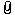Tangent to curvesFrom: bemfarmer 7 Mar 2013  (1 of 4)Is there a way to find the tangent Point, between a curve, and a given vector indicated by a line? (or a script.) For example, the minimum of the curve, indicated by a horizontal vector (line), near the red arrow. Or for example, the rightmost point of the curve, indicated by the vertical vector (line), near the other red arrow. (Placing two tan-tan circles between a line and the curve, at the two outer points where the radius gets minimized, narrows down the tangent point.)Attachments:ShellZcurve07.3dmShellZcurve07.png